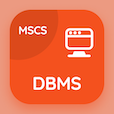Online CS Degree Courses

Digital Logic Design MCQs

Digital Logic Design MCQ - Topics

Practice Carry Propagation Multiple Choice Questions (MCQ Quiz), Carry Propagation quiz answers PDF to study digital logic design course for digital logic design online classes. MSI and PLD Components Multiple Choice Questions and Answers (MCQs), Carry Propagation quiz questions for online college courses. Carry Propagation Book PDF: introduction to msi and pld components, decoders and encoders, decimal adder, magnitude comparator test prep for top computer science schools.

"Carry generator in full adder has expression i.e." MCQ PDF: carry propagation App APK with g=ab, g=a+b, g=a-b, and g=a\b choices for online college courses. Learn carry propagation quiz questions for merit scholarship test and certificate programs for computer science associate degree.

## MCQ on Carry Propagation Quiz

MCQ: Carry generator in full adder has expression i.e.

G=AB
G=A+B
G=A-B
G=A\B

MCQ: The limiting factor on a speed of parallel adder is

input delay
carry propagation delay
input propagation delay
output delay

MCQ: The problem of carrying propagation delay was resolved by

MCQ: The time takes to carry and propagate through full adder is known as

cycle delay
clock delay
propagation delay
reset delay

MCQ: Carry propagate in full adder has expression i.e.

A AND B
A OR B
A XOR B
A NAND B

### More Quizzes on Digital Logic Design Book